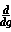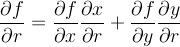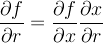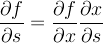# Math2.org Math Tables: Differentiation Identities(Math)(Fundamental Theorem for Derivatives)c f(x) = cf(x) (c is a constant)(f(x) + g(x)) =f(x) +g(x)f(g(x)) =f(g) *g(x) (chain rule)f(x)g(x) = f' (x)g(x) + f(x)g '(x) (product rule)

### Partial Differentiation Identities

if f( x(r,s), y(r,s) )if f( x(r,s) )